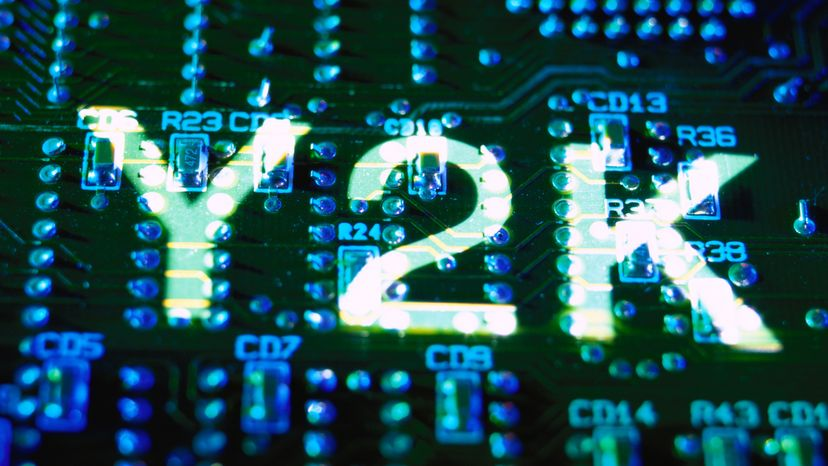# What is the Year 2038 problem?Year 2038 is a common problem for programs written in C programming language. Lawrence Lawry / Getty Images

The Year 2000 problem is understood by most people these days because of the large amount of media attention it received.

Most programs written in the C programming language are relatively immune to the Y2K problem, but suffer instead from the Year 2038 problem. This problem arises because most C programs use a library of routines called the standard time library . This library establishes a standard 4-byte format for the storage of time values, and also provides a number of functions for converting, displaying and calculating time values.

The standard 4-byte format assumes that the beginning of time is January 1, 1970, at 12:00:00 a.m. This value is 0. Any time/date value is expressed as the number of seconds following that zero value. So the value 919642718 is 919,642,718 seconds past 12:00:00 a.m. on January 1, 1970, which is Sunday, February 21, 1999, at 16:18:38 Pacific time (U.S.). This is a convenient format because if you subtract any two values, what you get is a number of seconds that is the time difference between them. Then you can use other functions in the library to determine how many minutes/hours/days/months/years have passed between the two times.

If you have read How Bits and Bytes Work, you know that a signed 4-byte integer has a maximum value of 2,147,483,647, and this is where the Year 2038 problem comes from. The maximum value of time before it rolls over to a negative (and invalid) value is 2,147,483,647, which translates into January 19, 2038. On this date, any C programs that use the standard time library will start to have problems with date calculations.

This problem is somewhat easier to fix than the Y2K problem on mainframes, fortunately. Well-written programs can simply be recompiled with a new version of the library that uses, for example, 8-byte values for the storage format. This is possible because the library encapsulates the whole time activity with its own time types and functions (unlike most mainframe programs, which did not standardize their date formats or calculations). So the Year 2038 problem should not be nearly as hard to fix as the Y2K problem was.